PRINTABLE FOR KIDS

XII (12) HSC

XI (11) FYJC
X (10) SSC

### Practice Set 6.2 Statistics Class 10th Mathematics Part 1 MHB Solution

Practice Set 6.2

1. The following table shows classification of number of workers and the number of hours…
2. The frequency distribution table shows the number of mango trees in a grove and their…
3. The following table shows the classification of number of vehicles and their speeds on…
4. The production of electric bulbs in different factories is shown in the following…

###### Practice Set 6.2

Question 1.

The following table shows classification of number of workers and the number of hours they work in a software company. Find the median of the number of hours they work.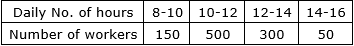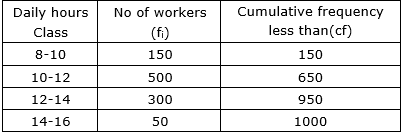⇒ N = 1000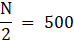⇒ 500 Lies in class 10-12

⇒ Median class 10-12

L = lower limit of median class = 10

N = sum of frequencies = 1000

h = class interval of median class = 2

f = frequency of median class = 500

cf = cumulative frequency of class preceding median class = 150

⇒ Median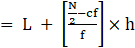⇒ Median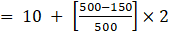⇒ Median = 11.4

Question 2.

The frequency distribution table shows the number of mango trees in a grove and their yield of mangoes. Find the median of data.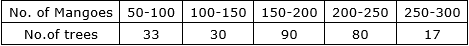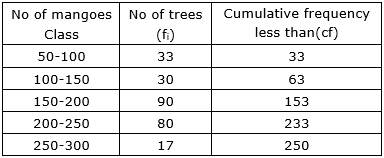⇒ N = 250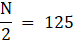⇒ 125 Lies in class 100-150

⇒ Median class 100-150

L = lower limit of median class = 100

N = sum of frequencies = 250

h = class interval of median class = 50

f = frequency of median class = 30

cf = cumulative frequency of class preceding median class = 33

⇒ Median⇒ Median =⇒ Median = 253.33

Question 3.

The following table shows the classification of number of vehicles and their speeds on Mumbai-Pune express way. Find the median of the data.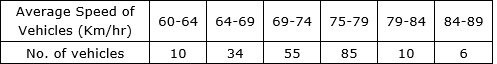The class is discontinuous between 69-74 and 75-79

Converting the to continuous class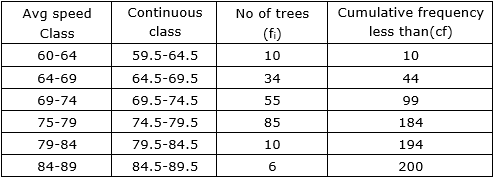⇒ N = 200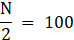⇒ 100 Lies in class 74.5-79.5

⇒ Median class 74.5-79.5

L = lower limit of median class = 74.5

N = sum of frequencies = 200

h = class interval of median class = 5

f = frequency of median class = 85

cf = cumulative frequency of class preceding median class = 99

⇒ Median⇒ Median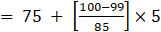⇒ Median = 74.558

Question 4.

The production of electric bulbs in different factories is shown in the following table. Find the median of the productions.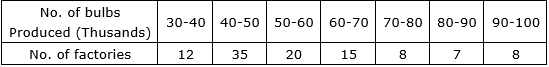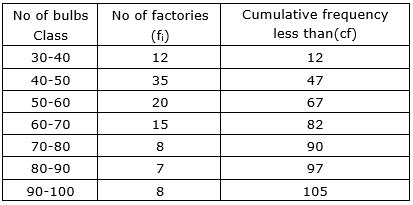⇒ N = 105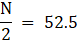⇒ 52.5 Lies in class 50-60

⇒ Median class 50-60

L = lower limit of median class = 50

N = sum of frequencies = 105

h = class interval of median class = 10

f = frequency of median class = 20

cf = cumulative frequency of class preceding median class = 47

⇒ Median⇒ Median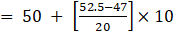⇒ Median = 52.75

⇒ In thousands = 52.75× 1000 = 52750 lamps

## PDF FILE TO YOUR EMAIL IMMEDIATELY PURCHASE NOTES & PAPER SOLUTION. @ Rs. 50/- each (GST extra)

SUBJECTS

HINDI ENTIRE PAPER SOLUTION

MARATHI PAPER SOLUTION
SSC MATHS I PAPER SOLUTION
SSC MATHS II PAPER SOLUTION
SSC SCIENCE I PAPER SOLUTION
SSC SCIENCE II PAPER SOLUTION
SSC ENGLISH PAPER SOLUTION
SSC & HSC ENGLISH WRITING SKILL
HSC ACCOUNTS NOTES
HSC OCM NOTES
HSC ECONOMICS NOTES
HSC SECRETARIAL PRACTICE NOTES

2019 Board Paper Solution

HSC ENGLISH SET A 2019 21st February, 2019

HSC ENGLISH SET B 2019 21st February, 2019

HSC ENGLISH SET C 2019 21st February, 2019

HSC ENGLISH SET D 2019 21st February, 2019

SECRETARIAL PRACTICE (S.P) 2019 25th February, 2019

HSC XII PHYSICS 2019 25th February, 2019

CHEMISTRY XII HSC SOLUTION 27th, February, 2019

OCM PAPER SOLUTION 2019 27th, February, 2019

HSC MATHS PAPER SOLUTION COMMERCE, 2nd March, 2019

HSC MATHS PAPER SOLUTION SCIENCE 2nd, March, 2019

SSC ENGLISH STD 10 5TH MARCH, 2019.

HSC XII ACCOUNTS 2019 6th March, 2019

HSC XII BIOLOGY 2019 6TH March, 2019

HSC XII ECONOMICS 9Th March 2019

SSC Maths I March 2019 Solution 10th Standard11th, March, 2019

SSC MATHS II MARCH 2019 SOLUTION 10TH STD.13th March, 2019

SSC SCIENCE I MARCH 2019 SOLUTION 10TH STD. 15th March, 2019.

SSC SCIENCE II MARCH 2019 SOLUTION 10TH STD. 18th March, 2019.

SSC SOCIAL SCIENCE I MARCH 2019 SOLUTION20th March, 2019

SSC SOCIAL SCIENCE II MARCH 2019 SOLUTION, 22nd March, 2019

XII CBSE - BOARD - MARCH - 2019 ENGLISH - QP + SOLUTIONS, 2nd March, 2019

HSC Maharashtra Board Papers 2020

(Std 12th English Medium)

HSC ECONOMICS MARCH 2020

HSC OCM MARCH 2020

HSC ACCOUNTS MARCH 2020

HSC S.P. MARCH 2020

HSC ENGLISH MARCH 2020

HSC HINDI MARCH 2020

HSC MARATHI MARCH 2020

HSC MATHS MARCH 2020

SSC Maharashtra Board Papers 2020

(Std 10th English Medium)

English MARCH 2020

HindI MARCH 2020

Hindi (Composite) MARCH 2020

Marathi MARCH 2020

Mathematics (Paper 1) MARCH 2020

Mathematics (Paper 2) MARCH 2020

Sanskrit MARCH 2020

Important-formula

THANKS